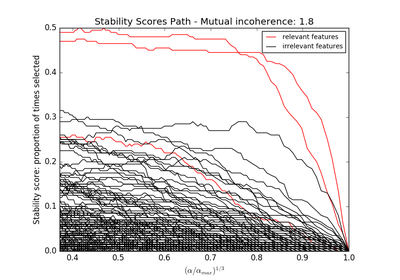# `sklearn.linear_model`.lasso_stability_path¶

`sklearn.linear_model.``lasso_stability_path`(X, y, scaling=0.5, random_state=None, n_resampling=200, n_grid=100, sample_fraction=0.75, eps=8.8817841970012523e-16, n_jobs=1, verbose=False)[source]

Stability path based on randomized Lasso estimates

Read more in the User Guide.

Parameters: X : array-like, shape = [n_samples, n_features] training data. y : array-like, shape = [n_samples] target values. scaling : float, optional, default=0.5 The alpha parameter in the stability selection article used to randomly scale the features. Should be between 0 and 1. random_state : integer or numpy.random.RandomState, optional The generator used to randomize the design. n_resampling : int, optional, default=200 Number of randomized models. n_grid : int, optional, default=100 Number of grid points. The path is linearly reinterpolated on a grid between 0 and 1 before computing the scores. sample_fraction : float, optional, default=0.75 The fraction of samples to be used in each randomized design. Should be between 0 and 1. If 1, all samples are used. eps : float, optional Smallest value of alpha / alpha_max considered n_jobs : integer, optional Number of CPUs to use during the resampling. If ‘-1’, use all the CPUs verbose : boolean or integer, optional Sets the verbosity amount alphas_grid : array, shape ~ [n_grid] The grid points between 0 and 1: alpha/alpha_max scores_path : array, shape = [n_features, n_grid] The scores for each feature along the path.

Notes

See examples/linear_model/plot_sparse_recovery.py for an example.

## Examples using `sklearn.linear_model.lasso_stability_path`¶Sparse recovery: feature selection for sparse linear models